# Nonagon

Regular nonagon (enneagon)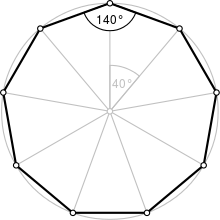A regular nonagon (enneagon)
Type Regular polygon
Edges and vertices 9
Schläfli symbol {9}
Coxeter diagramSymmetry group Dihedral (D9), order 2×9
Internal angle (degrees) 140°
Dual polygon Self
Properties Convex, cyclic, equilateral, isogonal, isotoxal

In geometry, a nonagon /ˈnɒnəɡɒn/ (or enneagon /ˈɛnəɡɒn/) is a nine-sided polygon or 9-gon.

The name "nonagon" is a prefix hybrid formation, from Latin (nonus, "ninth" + gonon), used equivalently, attested already in the 16th century in French nonogone and in English from the 17th century. The name "enneagon" comes from Greek enneagonon (εννεα, "nine" + γωνον (from γωνία = "corner")), and is arguably more correct, though somewhat less common than "nonagon".

## Regular nonagon

A regular nonagon is represented by Schläfli symbol {9} and has internal angles of 140°. The area of a regular nonagon of side length a is given bywhere the radius r of the inscribed circle of the regular nonagon isand where R is the radius of its circumscribed circle:## Construction

Although a regular nonagon is not constructible with compass and straightedge (as 9 = 32, which is not a product of distinct Fermat primes), there are very old methods of construction that produce very close approximations.

It can be also constructed using neusis, or by allowing the use of an angle trisector.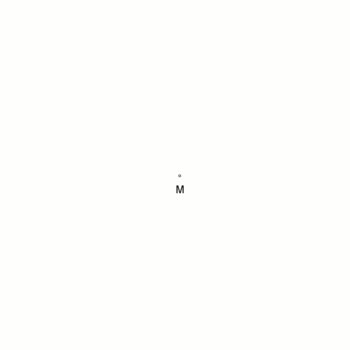Nonagon, an animation from a neusis construction based on the angle trisection 120° by means of the Tomahawk

The following is an approximate construction of a nonagon using a straightedge and compass.

Example to illustrate the error, when the constructed central angle is 39.99906°:
At a circumscribed circle radius r = 100 m, the absolute error of the 1st side would be approximately 1.6 mm.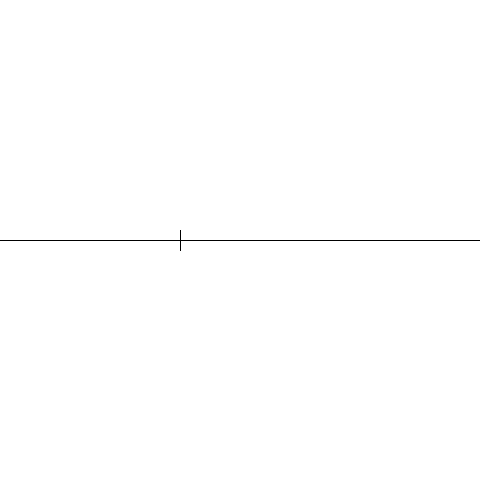Another two animations of an approximate construction

• Downsize the angle JMK (also 60°) with four bisections of angle and make a thirds of circular arc MON with an approximate solution between bisections of angle w3 and w4.
• Straight auxiliary line g aims over the point O to the point N (virtually a ruler at the points O and N applied), between O and N, therefore no auxiliary line.
Thus, the circular arc MON is freely accessible for the later intersection point R.RMK = 40.0000000052441...°
360° ÷ 9 = 40°RMK - 40° = 5.2...E-9°
Example to illustrate the error:
r = 100,000 km, the absolute error of the 1st side would be approximately 8.6 mm.Nonagon at a given circumscribed circle, animationNonagon at a given side length, animation

## Symmetry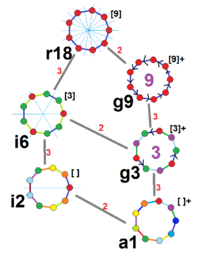Symmetries of a regular enneagon. Vertices are colored by their symmetry positions. Blue mirrors are drawn through vertices, and purple mirrors are drawn through edge. Gyration orders are given in the center.

The regular enneagon has Dih9 symmetry, order 18. There are 2 subgroup dihedral symmetries: Dih3 and Dih1, and 3 cyclic group symmetries: Z9, Z3, and Z1.

These 6 symmetries can be seen in 6 distinct symmetries on the enneagon. John Conway labels these by a letter and group order. Full symmetry of the regular form is r18 and no symmetry is labeled a1. The dihedral symmetries are divided depending on whether they pass through vertices (d for diagonal) or edges (p for perpendiculars), and i when reflection lines path through both edges and vertices. Cyclic symmetries in the middle column are labeled as g for their central gyration orders.

Each subgroup symmetry allows one or more degrees of freedom for irregular forms. Only the g9 subgroup has no degrees of freedom but can seen as directed edges.

## Graphs

The K9 complete graph is often drawn as a regular enneagon with all 36 edges connected. This graph also represents an orthographic projection of the 9 vertices and 36 edges of the 8-simplex.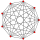8-simplex (8D)

## Pop culture references

They Might Be Giants have a song entitled "Nonagon" on their children's album Here Come the 123s. It refers to both an attendee at a party at which "everybody in the party is a many-sided polygon" and a dance they perform at this party. Slipknot's logo is also a version of a nonagon, being a nine-pointed star made of three triangles. King Gizzard & the Lizard Wizard have an album titled 'Nonagon Infinity', the album art featuring a nonagonal complete graph.

## Architecture

Temples of the Baha'i Faith are required to be nonagonal.

The U.S. Steel Tower is an irregular nonagon.